## RS Aggarwal Class 7 Solutions Chapter 12 Simple Interest Ex 12B

These Solutions are part of RS Aggarwal Solutions Class 7. Here we have given RS Aggarwal Solutions Class 7 Chapter 12 Simple Interest Ex 12B.

Other Exercises

Question 1.
Solution:
Principal (P) = Rs. 6250
Rate of (R) = 4% p.a.
Period (T) = 6 months = $$\frac { 1 }{ 2 }$$ yearQuestion 2.
Solution:
Amount (A) = Rs. 3605
Rate (R) = 5% p.a.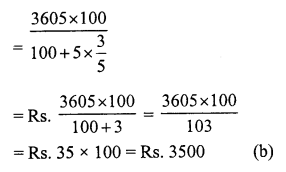Question 3.
Solution:
Let sum (P) = Rs. 100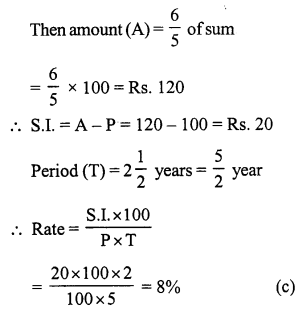Question 4.
Solution:
Principal (P) = Rs. 8000
Amount (A) – Rs. 8360
S.I. = A – P = Rs. 8360 – Rs. 8000 = Rs. 360
Rate (R) = 6% p.a.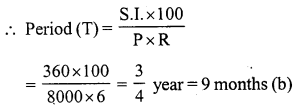Question 5.
Solution:
Let sum (P) = Rs. 100
Then amount (A) = Rs. 100 x 2 = Rs. 200
S.I. = A – P = Rs. 200 – Rs. 100 = Rs. 100
Period (T) = 10 years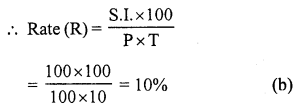Question 6.
Solution:
S.I. = Rs. x
Rate (R) = x% p.a.
Time (T) = x year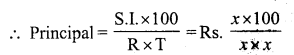Rs. $$\frac { 100 }{ x }$$ (c)

Question 7.
Solution:
Let sum (P) = Rs. 100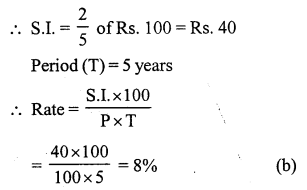Question 8.
Solution:
A’s principal (P) = Rs. 8000
Rate (R) = 12% p.a.
B’s principal = Rs. 9100
Rate = 10%
Let after x years, then amount will be equal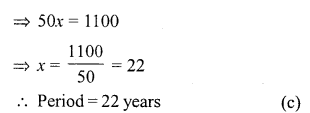Question 9.
Solution:
Amount (A) = Rs. 720
Principal (P) = Rs. 600
S.I. = A – P = Rs. 720 – 600 = Rs. 120
Period (T) = 4 years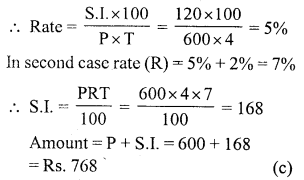Question 10.
Solution: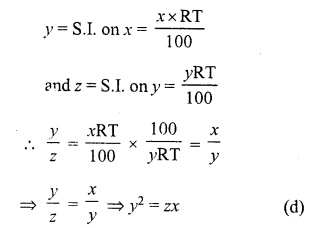Question 11.
Solution:
Let sum (P) = Rs. 100
Their S.I. = 0.125 of Rs. 100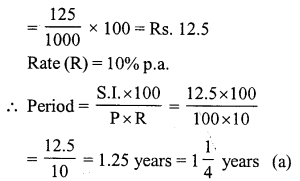Question 12.
Solution:
S.I. = Rs. 210Hope given RS Aggarwal Solutions Class 7 Chapter 12 Simple Interest Ex 12B are helpful to complete your math homework.

If you have any doubts, please comment below. Learn Insta try to provide online math tutoring for you.